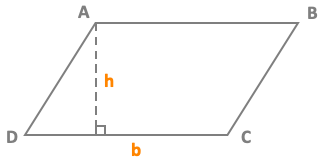# Area of a parallelogramArea A = b x h

DC = base = b
h = height

## Example

ABC is a parallelogram with a base b = 6 cm and a height h = 3 cm
Area A of a parallelogram ABCD = b x h = 6 x 3 = 18 cm²

## Calculate the area of a parallelogram

 Base b Height h Area A of the parallelogram

## Definition of a parallelogram

A parallelogram is a quadrilateral that has its opposite sides parallel.
A parallelogram is a special type of that has equal and parallel opposite sides.
A parallelogram is a quadrilateral that has two pairs of parallel sides.

A parallelogram is a quadrilateral that has two pairs of parallel sides.
A parallelogram is a quadrilateral, or four-sided shape, with two sets of parallel sides.
Area = Base x Height
The base is the length of the long, flat side on the bottom.
The height is the distance from the base straight up to its parallel side.

The area of a parallelogram can be calculated by finding the product of its base with the altitude.

## Properties of the parallelogram

In a parallelogram, the opposite sides are parallel and have the same length.
In a parallelogram, the diagonals have the same midpoint. The midpoint of the diagonals is the center of symmetry of the parallelogram.

In a parallelogram, the opposite angles have the same measure.
In a parallelogram, consecutive angles are supplementary.
If a quadrilateral has its opposite sides parallel, then that quadrilateral is a parallelogram.
If a quadrilateral has its diagonals which have the same midpoint, then this quadrilateral is a parallelogram.

The opposite or facing sides of a parallelogram are of equal length and the opposite angles of a parallelogram are of equal measure.
The opposite sides of a parallelogram are parallel to each other.
The opposite sides of a parallelogram are equal in length.
The opposite angles of a parallelogram are equal in measure.
Diagonals of a parallelogram bisect each other.